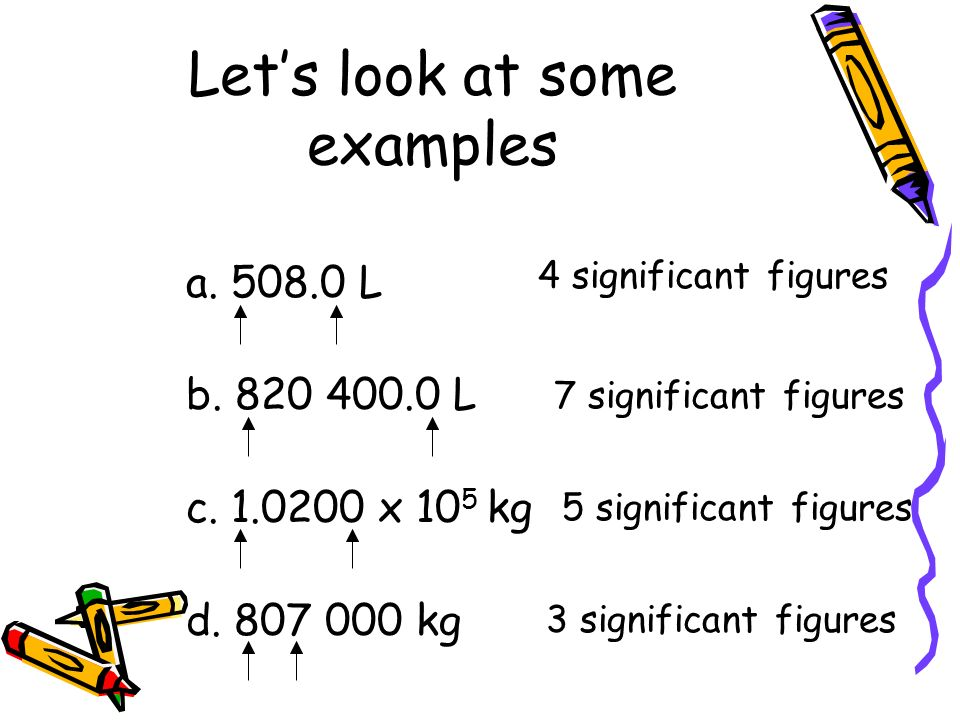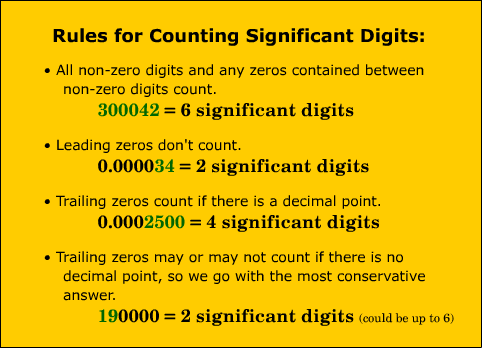# Significant Figures Multiplication Study Guide

INTRODUCTION

Now that “everybody” has a calculator that can calculate up to six or eight (or more) figures, we must understand that every time we have to take some measurements, going into that much accuracy could be a cumbersome process even frustrating sometimes. Imagine going to a milkman and asking him for 989.6745 ml of milk. No matter your intentions, it’s pretty certain that he will give you a weird look. In day-to-day life, this level of accuracy is neither needed nor practically possible. This significant figure helps us simplify these numbers.What are significant figures?

• To establish the number that is provided in the form of digits, significant figures are needed.
• These digits represent numbers in a meaningful way. Counting all the numbers starting with the first non-zero digit on the left will reveal the number of significant figures.
• These figures are accurate and required for expressing the quantities of a length, volume, mass, measurement, and so on.
• When calculating significant values, mathematical operations like addition, subtraction, multiplication, and division are utilized.

### RULES OF SIGNIFICANT FIGURES

There are several principles that must be observed and recalled while measuring the important numbers of a computed value.

1. Except for zero, all digits are always significant. 894621, for example, has six significant digits.
2. In between two non-zero numbers, any zeros are significant. 10.007, for instance, has five significant digits.
3. When zeros appear to the right-hand side of a decimal place and to the left-hand side of a non-zero number, they have no significance. 0.0012, for instance, has two significant digits.
4. It is significant if zeros are put to the right of the decimal, and there is no non-zero digit following that. 80.00, for example, has four significant digits.
5. When it comes to measurements, zeros positioned to the right of the final non-zero digit are significant. 3560 m, for instance, has four significant figures.Source

### WHEN IT COMES TO MULTIPLICATION, THE PRECEDING RULE APPLIES

• The number of significant figures in the response is determined by the least number of significant figures in any integer in the issue.
• This means that in order to apply this rule, you must be able to distinguish significant figures.

Example 1. 2.5 x 3.42

The solution to this issue is 8.6 (rounded up from the calculator reading of 8.55). Why?There are two significant figures in 2.5, but three in 3.42. Because two significant figures are less precise than three, the answer has two.

### SIGNIFICANT FIGURES MULTIPLICATION

• In multiplication, the principle that regulates the use of significant numbers is that the final outcome can only be as exact as the least precise measurement.
• Nevertheless, we tally the significant figures in each measurement rather than the number of decimal places in this scenario: When observations are multiplied, the result can only have the least accurate measurement as a significant figure.Source

## CONCLUSION:

1. To establish the number that is provided in the form of digits, significant figures are needed.
2. The number of significant figures in the response is determined by the least number of significant figures in any quantity in the question.

### FAQs:

1. What is the rule for sig figs when multiplying?

Use the following rule for multiplication and division: The number of significant figures in the response is determined by the least number of significant figures in any quantity in the question.

2. How do you do sig figs with multiple operations?

Retain at least one more significant figure in interim findings than you’ll need in your final solution when completing multi-step calculations. For instance, if a final result demands two significant figures, all calculations should use at least three significant figures.

3. What rule should be used if multiple operations are applied to determine the number of significant figures in a calculated result or measurement?

When multiplying or dividing, the rule would be that the final conclusion should get the same number of significant figures as the value with the fewest significant figures.

We hope you enjoyed studying this lesson and learned something cool about Significant figures in Multiplication! Join our Discord community to get any questions you may have answered and to engage with other students just like you! Don’t forget to download our App to experience our fun VR classrooms – we promise, it makes studying much more fun! 😎

## SOURCES:

1. Significant Figure Rules:http://www.ruf.rice.edu/~kekule/SignificantFigureRules1 Accessed 16 march 2022
2. Significant Figures:https://www.cuemath.com/numbers/significant-figures/ Accessed 16 march 2022
3. Significant Figures:https://byjus.com/maths/significant-figures/ Accessed 16 march 2022
]]>